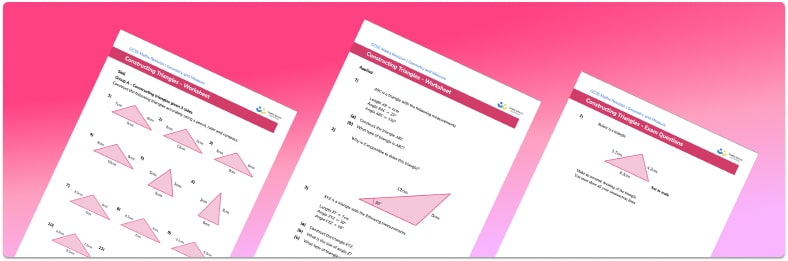# Constructing triangles worksheet• Section 1 of the constructing triangles worksheet contains 36 skills-based constructing triangles questions, in 3 groups to support differentiation
• Section 2 contains 4 applied constructing triangles questions with a mix of worded problems and deeper problem solving questions
• Section 3 contains 4 foundation and higher level GCSE exam style constructing triangles questions
• Answers and a mark scheme for all constructing triangles questions are provided
• Questions follow variation theory with plenty of opportunities for students to work independently at their own level
• All questions created by fully qualified expert secondary maths teachers
• Suitable for GCSE maths revision for AQA, OCR and Edexcel exam boards

• This field is for validation purposes and should be left unchanged.

You can unsubscribe at any time (each email we send will contain an easy way to unsubscribe). To find out more about how we use your data, see our privacy policy.

### Constructing triangles at a glance

Sometimes we need to construct accurate drawings of triangles. This can be done given certain information, using a ruler, protractor and a pair of compasses.

The information that we are given for triangle construction will vary depending on the situation. For example we might be told the length of each side (SSS), the size of two angles and the length of the straight line between them (ASA) or facts such as that the triangle is an isosceles triangle, equilateral triangle or right angle triangle.  The method and equipment that we use will depend on which measurements we are given.

As an example, let’s think about how to construct triangle ABC, where AB=10cm, BC=8cm and angle ABC=60o. We can first draw one side, AB which is 10cm long. Next we can measure the angle ABC using a protractor. Once we have the correct angle, we can measure the length of BC as 8cm. Finally, we can draw the third side of the triangle so that it joins the other two sides.

Looking forward, students can then progress to additional geometry worksheets, for example a congruent triangles worksheetFor more teaching and learning support on Geometry our GCSE maths lessons provide step by step support for all GCSE maths concepts.

## Do you have KS4 students who need more focused attention to succeed at GCSE?There will be students in your class who require individual attention to help them succeed in their maths GCSEs. In a class of 30, it’s not always easy to provide.

Help your students feel confident with exam-style questions and the strategies they’ll need to answer them correctly with our dedicated GCSE maths revision programme.

Lessons are selected to provide support where each student needs it most, and specially-trained GCSE maths tutors adapt the pitch and pace of each lesson. This ensures a personalised revision programme that raises grades and boosts confidence.

Find out more